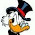## 17 September 2013

### 514. Extracting Frequency data from a gaussian 09 calculation for gnuplot

This is another python script.

Say you've done a computation along the lines of this:
```
#P rBP86/GEN 5D Pseudo(Read) Opt=() Freq=() SCF=(MaxCycle=999 ) Punch=(MO) Pop=()

```
and want the data in a neat data file, like this:
```
33.237 0.0023 0.0536
39.9976 0.0043 0.8305
69.7345 0.0129 0.3348
84.7005 0.0173 0.7027
[..]
3133.0068 6.2938 0.6114
3143.8021 6.3551 0.3775
3164.9242 6.4685 0.8829
3221.8787 6.6972 4.6005

```

Then you can use the following python (2.x) script, g09freq:

```#!/usr/bin/python
# Compatible with python 2.7
# Reads frequency output from a g09 (gaussian) calculation
# Usage ex.: g09freq g09.log ir.dat
import sys

def ints2float(integerlist):
for n in range(0,len(integerlist)):
integerlist[n]=float(integerlist[n])
return integerlist

def parse_in(infile):
g09output=open(infile,'r')
captured=[]
for line in g09output:
if ('Frequencies' in line) or ('Frc consts' in line) or ('IR Inten' in line):
captured+=[line.strip('\n')]
g09output.close()
return captured

def format_captured(captured):
vibmatrix=[]
steps=len(captured)
for n in range(0,steps,3):
freqs=ints2float(filter(None,captured[n].split(' '))[2:5])
forces=ints2float(filter(None,captured[n+1].split(' '))[3:6])
intensities=ints2float(filter(None,captured[n+2].split(' '))[3:6])
for m in range(0,3):
vibmatrix+=[[freqs[m],forces[m],intensities[m]]]
return vibmatrix

def write_matrix(vibmatrix,outfile):
f=open(outfile,'w')
for n in range(0,len(vibmatrix)):
item=vibmatrix[n]
f.write(str(item)+'\t'+str(item)+'\t'+str(item)+'\n')
f.close()
return 0

if __name__ == "__main__":
infile=sys.argv
outfile=sys.argv

captured=parse_in(infile)

if len(captured)%3==0:
vibmatrix=format_captured(captured)
else:
print 'Number of elements not divisible by 3 (freq+force+intens=3)'
exit()
success=write_matrix(vibmatrix,outfile)
if success==0:
print 'Read %s, parsed it, and wrote %s'%(infile,outfile)

```

Run it as e.g.
`g09freq g09.log test.out`

The output is compatible with gnuplot:
```gnuplot> set xrange [3500:0]
gnuplot> set yrange [10:-1]
gnuplot> plot './test.out' u 1:2 w impulse
```

1.Hi! I'm a beginner in G09 and I found very useful your script; however, I would like to know if you have already tested your script? I am asking you that because my log file has 36 frequencies, but the output from the script only contains 24 data. I would really appreciate your help. Thanks

1.You're right -- there's a small error with big repercussions:

for m in range(0,2):
vibmatrix+=[[freqs[m],forces[m],intensities[m]]]

should be

for m in range(0,3):
vibmatrix+=[[freqs[m],forces[m],intensities[m]]]

Otherwise it keeps ignoring the last frequency on each line in the output.

2.Hi,

I think using awk script will make this cleaner.
This is what I would have used to produce the same output.

==========================================
#!/bin/awk -f

/Frequencies/ { for (i=3;i<=NF; i++) { im++; freq[im ]=\$i} }
/Frc consts/ { for (i=NF;i>=4; i--) fc[im-(NF-i)]=\$i }
/IR Inten/ { for (i=NF;i>=4; i--) ir[im-(NF-i)]=\$i }

END { for (i=1;i<=im;i++) print freq[i],fc[i],ir[i] }
==========================================

3.